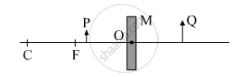# Consider the following diagram in which M is a mirror and P is an object and Q is its magnified image formed by the mirror. - Science

Consider the following diagram in which M is a mirror and P is an object and Q is its magnified image formed by the mirror.State the type of the mirror M and one characteristic property of the image Q.

#### Solution

The given mirror M is a concave spherical mirror. The characteristic property of the image Q is that it is virtual.

Concept: Concave Mirror
Is there an error in this question or solution?